# MathWorks Logo, Part Two. Finite Differences2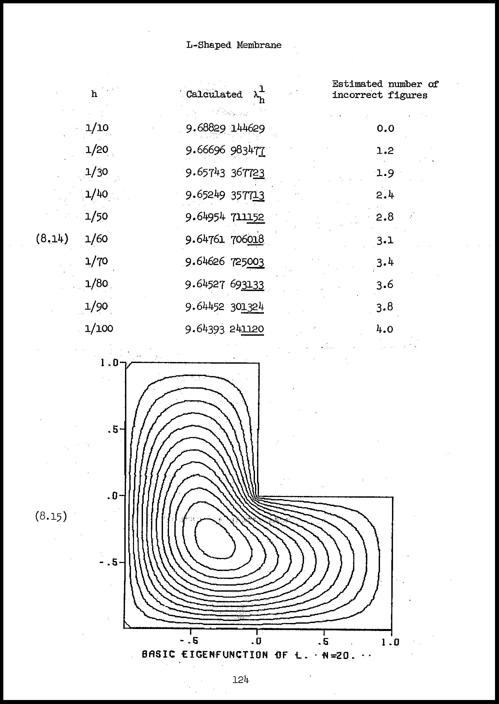After reviewing the state of affairs fifty years ago, I use classic finite difference methods, followed by extrapolation, to find the first eigenvalue of the region underlying the MathWorks logo.... 続きを読む >>

# MathWorks Logo, Part One. Why Is It L Shaped?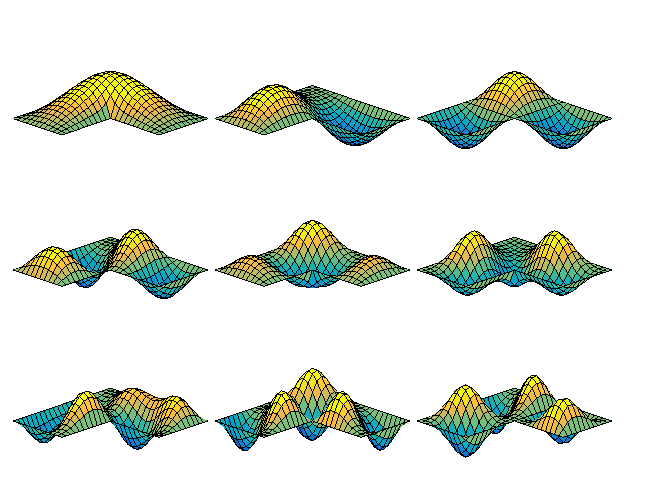MathWorks is the only company in the world whose logo satisfies a partial differential equation. Why is the region for this equation shaped like a capital letter L? ... 続きを読む >>

# Ordinary Differential Equations, Stiffness3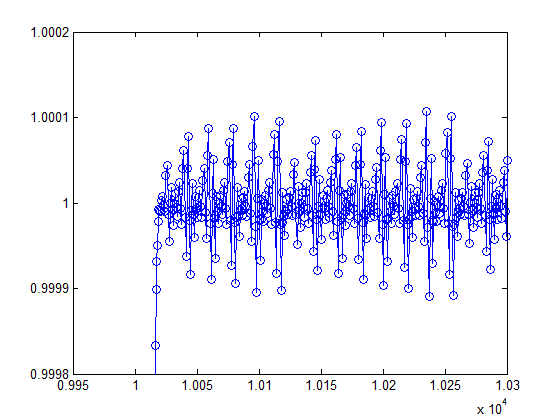Stiffness is a subtle concept that plays an important role in assessing the effectiveness of numerical methods for ordinary differential equations. (This article is adapted from section 7.9, "Stiffness", in Numerical Computing with MATLAB.) ... 続きを読む >>

# Ordinary Differential Equation Solvers ODE23 and ODE454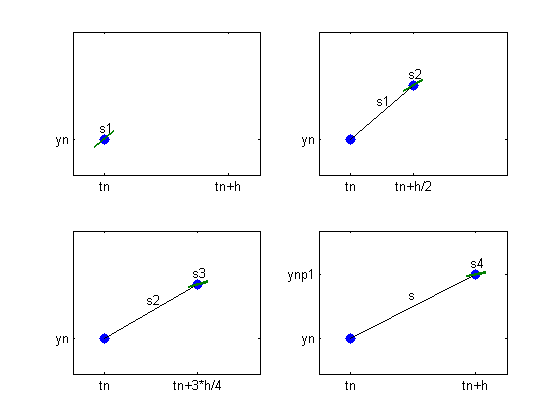The functions ode23 and ode45 are the principal MATLAB and Simulink tools for solving nonstiff ordinary differential equations.... 続きを読む >>

# Ordinary Differential Equation Suite4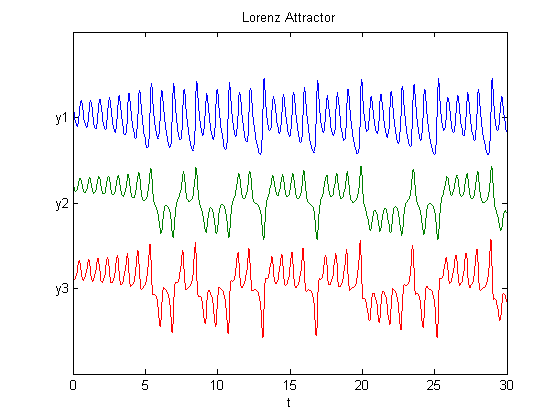MATLAB and Simulink have a powerful suite of routines for the numerical solution of ordinary differential equations. Today's post offers an introduction. Subsequent posts will examine several of the routines in more detail.... 続きを読む >>

# Periodic Solutions to the Lorenz Equations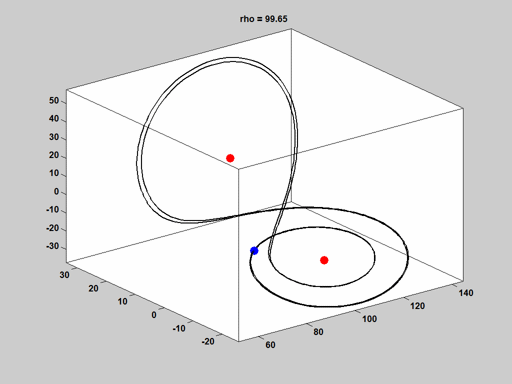Changing the value of a parameter in the equations that produce the famous Lorenz chaotic attractor yields nonlinear ordinary differential equations that have periodic solutions. ... 続きを読む >>